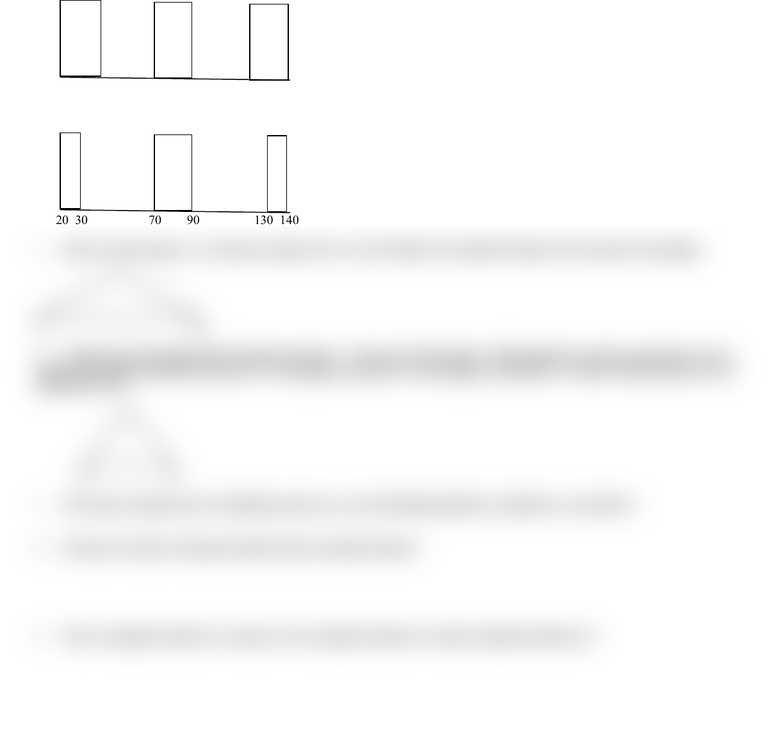Study Guides (380,000)
US (220,000)
UC-Irvine (3,000)
ECON (300)
Midterm

# ECON 15A Study Guide - Midterm Guide: Standard Deviation, Kurtosis, Box PlotExam

Department
Economics
Course Code
ECON 15A
Professor
Paul Shirey
Study Guide
Midterm

This preview shows half of the first page. to view the full 2 pages of the document.NAME: _________________________________ I.D.: _____________________________________
TEST TWO
PROBABILITY AND STATISTICS, ECON 15A
OCTOBER 21, 2016
1. Explain why the standard deviation, besides a measure of width, can also be thought of as a measure of error.
2. Estimate the standard deviation of the height of male UCI students. Explain why you picked that number.
3. Make an educated guess as to what the IQR is for the below graph.
20 40 70 90 120 140
4. Make an educated guess as to what the standard deviation is for the below graph.
20 30 70 90 130 140
5. Make an educated guess as to what percentage of the scores fall within two standard deviations of the mean for below graph.
0 50
6. Compare the below graph with the graph in question 5. The answer this question: Which graph has a greater percentage of scores
within one standard deviation of the mean? A) The graph in Question 5; B) The graph in Question 6; C) They are both the same; D) It is
impossible to tell.
14 17
7. If the index of dispersion of a distribution equals zero, is the distribution platykurtic, leptokurtic, or mesokurtic?
8. How does the Index of Dispersion differ from the standard deviation?
9. Why is it helpful to divide the covariance by the standard deviation of x and the standard deviation of y?